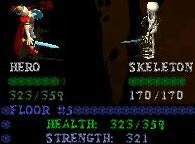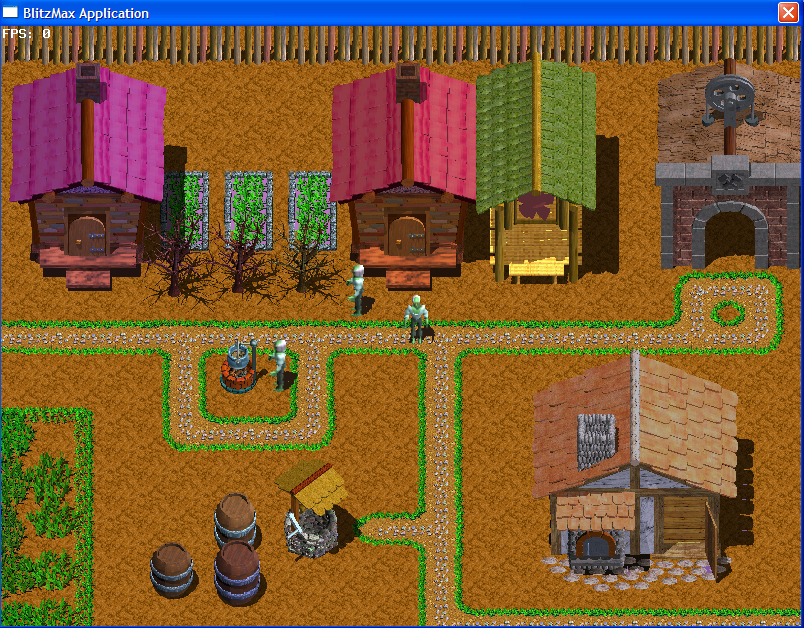-=+=- -=+=- -=+=- -=+=- -=+=- -=+=- -=+=- -=+=- -=+=- -=+=- -=+=- -=+=- -=+=- -=+=- -=+=- -=+=- -=+=- -=+=- -=+=- -=+=- -=+=- -=+=- -=+=- -=+=- -=+=- -=+=- -=+=- -=+=- -=+=- -=+=- (c) WidthPadding Industries 1987 0|265|0 -=+=- -=+=- -=+=- -=+=- -=+=- -=+=- -=+=- -=+=- -=+=- -=+=- -=+=- -=+=- -=+=- -=+=- -=+=- -=+=- -=+=- -=+=- -=+=- -=+=- -=+=- -=+=- -=+=- -=+=- -=+=- -=+=- -=+=- -=+=- -=+=- -=+=- SoCoder -> Showcase Home -> Role Playing Spell casting and big dragons. And maybe even some that aren't set in medieval times. Older -->Roguelike Explorer Simple Html5 exploration game with Touch support Pakz , Monkey Browser BasedDungeon Runes A text-RPG curtastic , Blitz Max WindowsPlayer vs Zombies or Armed Soldiers Fight in dungeons against monsters/soldiers Pakz , Monkey-X Html5 + keyboard Saviors of Gundthor The evil mage Arcanus has taken control of Gundthor! Save it. CodersRule Java WindowsGreenie's Mini Quest Join Greenie on a dungeon-romping adventure! Jayenkai Monkey Cross PlatformRPGMAKER3D - open source my never finished rpg maker 3d realtime BlitzWW191: Kinky Monster Dungeon The KING has been stolen by SANTA! Help for to get back at him for Princess DONALD! Evil Roy Ferguson BlitzDungeon Maker Classical dungeon crawler game in full 3D Wishbone Blitz WindowsEat Brains Fantasy RPG curtastic , Blitz Max WindowsMapEdit Abandoned tilemap editor flying_cucco Cobra Windows Older -->